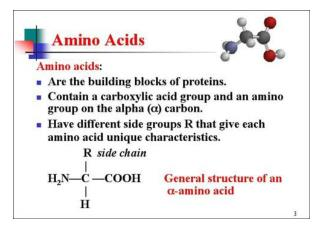DownloadDownload PresentationAmino Acids

# Amino Acids

Télécharger la présentation## Amino Acids

- - - - - - - - - - - - - - - - - - - - - - - - - - - E N D - - - - - - - - - - - - - - - - - - - - - - - - - - -
##### Presentation Transcript

1. Amino Acids

2. Amino Acids

3. Amino Acids

4. Amino Acids

5. Properties of Amino Acids • Ques. 4: Use the above structures to answer the questions below: • a. Which amino acid is most polar? • b. Which amino acid is least polar? • c. Which amino acid has an acidic side chain? • d. Which amino acid has a basic side chain?

6. Properties of Amino Acids • Ques. 5: Use the below structures to answer the questions: • a. Which amino acid is most polar? • b. Which amino acid is least polar? • c. Which amino acid is the most polar looking at thr and ser? • d. Which amino acid is the least polar looking at asp and glu?

7. Amino Acids • Zwitterion - acid and amine react with each other internally.

8. Amino Acids • -> -> Increasing pH -> -> • pH << pK << pH • Acids: HA molecular A- ionic • Bases: BH+ ionic B molecular

9. Amino Acids • -> -> Increasing pH -> -> • pH << pK << pH • Acids: HA molecular A- ionic • Bases: BH+ ionic B molecular

10. Amino Acids • -> -> Increasing pH -> -> • pH << pK << pH • Acids: HA molecular A- ionic • Bases: BH+ ionic B molecular

11. Amino Acids

12. Separations of Amino Acids

13. Amino Acids • -> -> Increasing pH -> -> • pH << pK << pH • Acids: HA molecular A- ionic • Bases: BH+ ionic B molecular • Mixture of val pI = 6; asp pI = 2.8, lys pI = 9.7in a buffer at pH = 6. What is the form of each?

14. Reactions Amino Acids - Salt Formation • Acid + amine --> salt (acid donates H+ to amine)

15. Reactions Amino Acids - Disulfide • 2 thiols + (O) --> disulfide + HOH

16. Synthesis of a peptide • Amine + acid --> amide + HOH

17. Synthesis of a peptide • Amine + acid --> amide + HOH

18. Synthesis of a peptide • Write repeating units of backbone and add side chains